This toolbox performs several different analyses on the local field potentials (LFP) generated from multichannel recording electrodes. After performing spike sorting the LFP of a channel can be acquired by low-pass filtering the recorded data. This then allows the relationship between the LFP and neural spiking to be investigated.

### Phase Locking

Phase locking describes the relationship between the timing of neural spiking and the phase of the LFP, at a given frequency. If a neuron is phase locked it means it has a preferred phase angle at which it is more likely to fire. If the neuron is not phase locked it fires more uniformly across all of the phase angles.

By using a continuous wavelet transform we can determine the phase of the LFP at a given frequency as a function of time allowing us to identify the phase of the LFP at each spike time of a specified neuron. From which we can build a distribution of the phase angles for the neurons.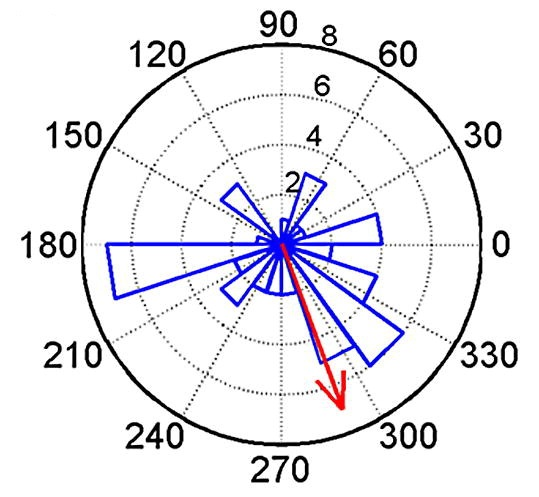Example distribution for a phase locked neuron, red arrow represents the preferred angle.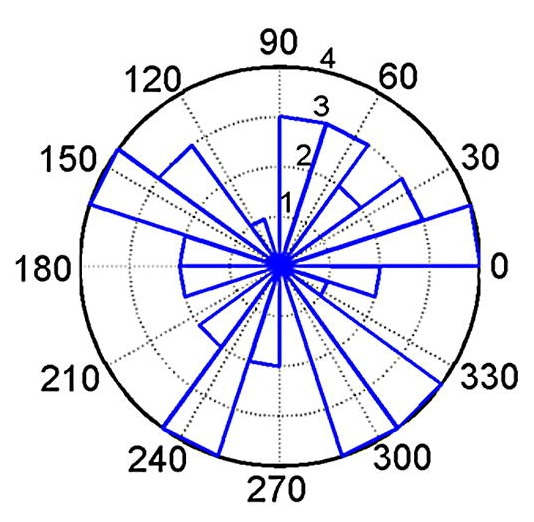Example distribution for a non phase locked neuron with no preferred angle.

By calculating the circular mean of the distribution of the phase angles and testing for the deviation from uniformity using a Rayleigh test we can determine if the neuron is phase locked or not. This can be repeated for different frequencies to determine which frequency or frequency band has a stronger relationship with the neuron spike timing.

There is also an option in the toolbox to calculate the phase locking with a time offset between the neuron spike action potential and the LFP to allow analysis of whether the LFP leads or lags the action potential.

The effects of either increased  or disrupted  phase locking our results have shown have an impact on learning and memory.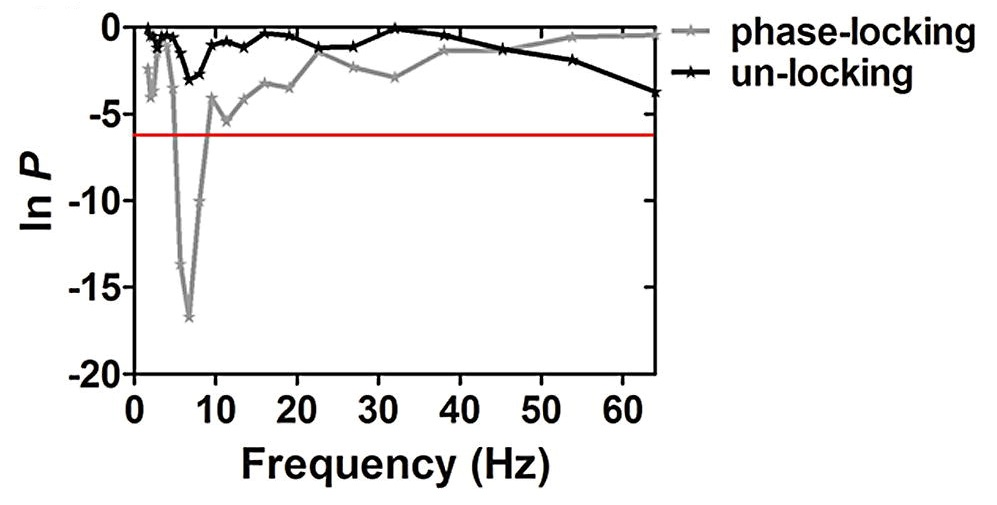Examples of significance of phase locking across frequency for two different neurons.

### Spike-Field Coherence

Similarly to phase locking the spike-field coherence (SFC) is a relationship between the neural spiking action potentials and the phase of the local field potential. SFC measures the phase synchronization between the action potentials and field potential oscillations.

To compute the SFC for each spike a segment of the LFP data centered on the spike is extracted. These sections are then averaged to calculate the spike-triggered average (STA). The frequency spectrum of the STA (fSTA) can then be calculated. In the toolbox this is achieved using multitaper analysis to give estimates of the PSD. The same method is then used to calculate the frequency spectra of each of the segments of LFP data individually the average of which is the spike triggered power (STP(f)).

Finally the spike field coherence is the fSTA over the STP(f) expressed as a percentage.

Similarly to the phase locking our results have shown changes in SFC specifically in the theta frequency band have impacts on learning and memory , .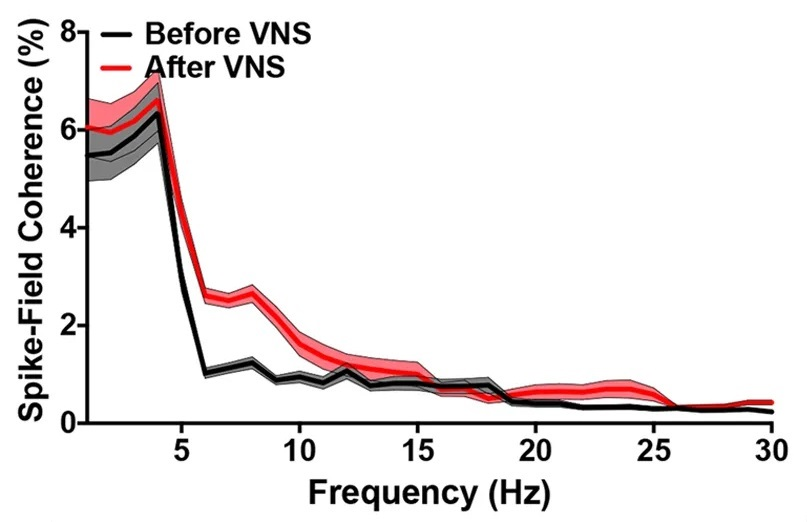Examples of spike field coherence pre and post vagal nerve stimulation.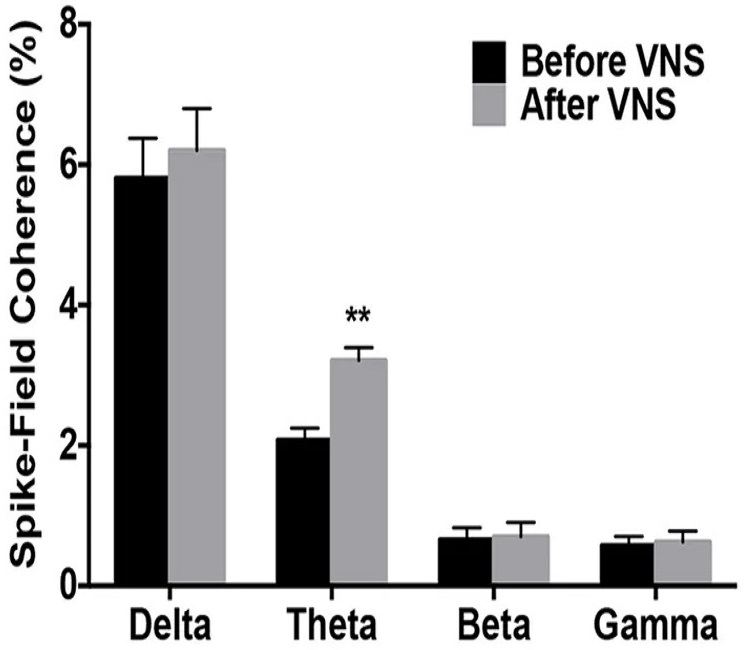Example of changes in the spike field coherence in different frequency bands pre and post vagal nerve stimulation.

### Amplitude Cross-Correlation

The amplitude cross-correlation is a relationship between two different LFPs, often between two different regions of the brain.

To calculate the amplitude cross-correlation both sets of LFP data are filtered into the frequency band of interest. From this the instantaneous amplitude is calculated using the Hilbert transform. The cross-correlation of the instantaneous amplitude is then calculated and the lag at which the maximum correlation occurs determined.

To ensure the significance of the results a bootstrap procedure is used, shifting the data by a random amount and repeating to allow a confidence interval to be determined. If the result from the original cross-correlation is greater than the 95% confidence interval from the bootstrapped values then the result is considered significant.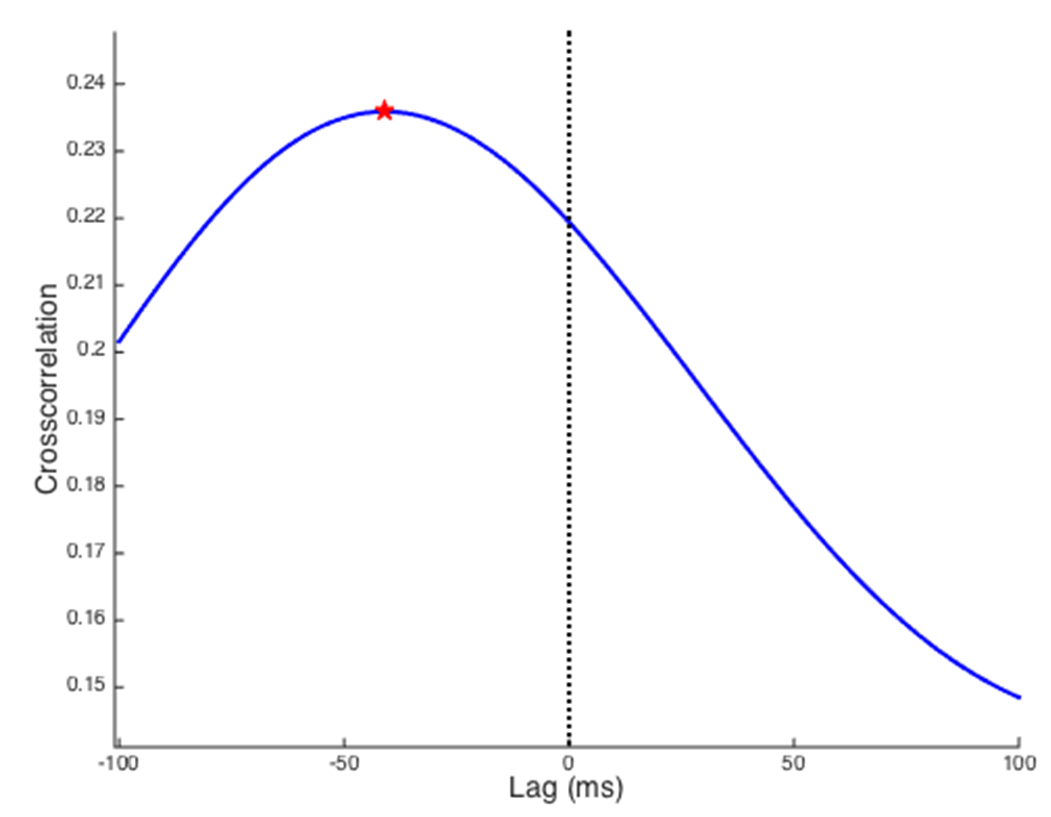Example of amplitude cross-correlation in the theta frequency band (peak marked with red star).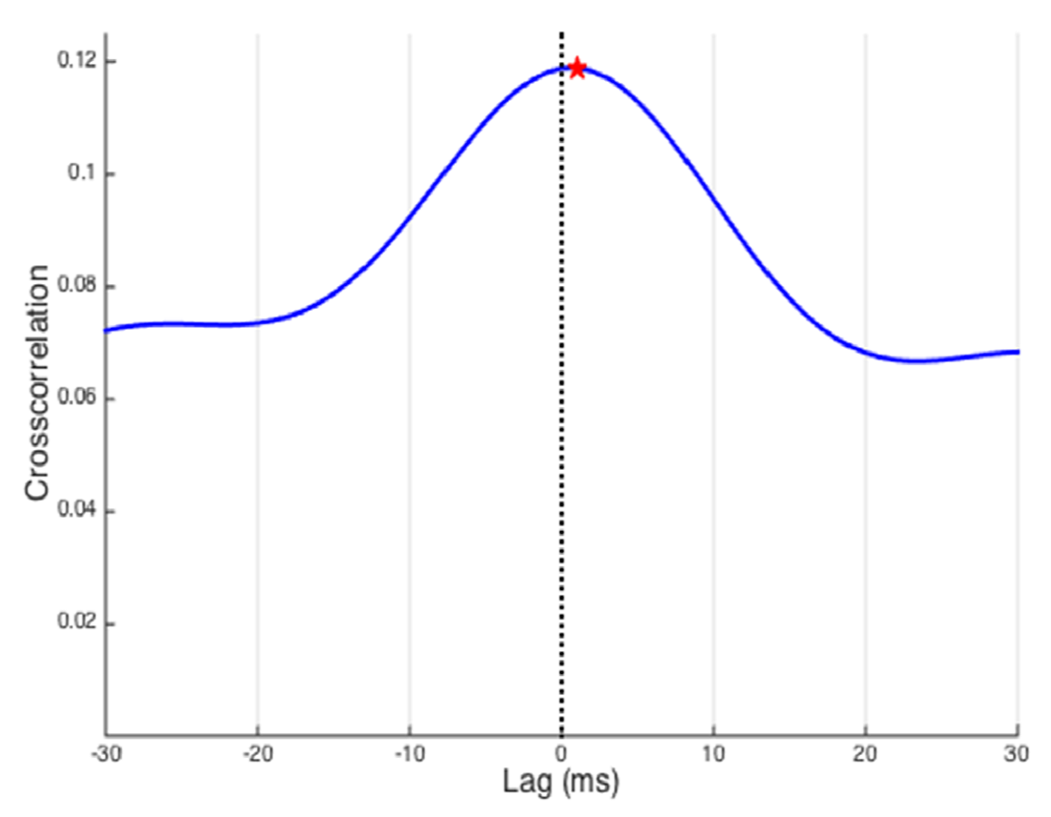Example of amplitude cross-correlation in the gamma frequency band.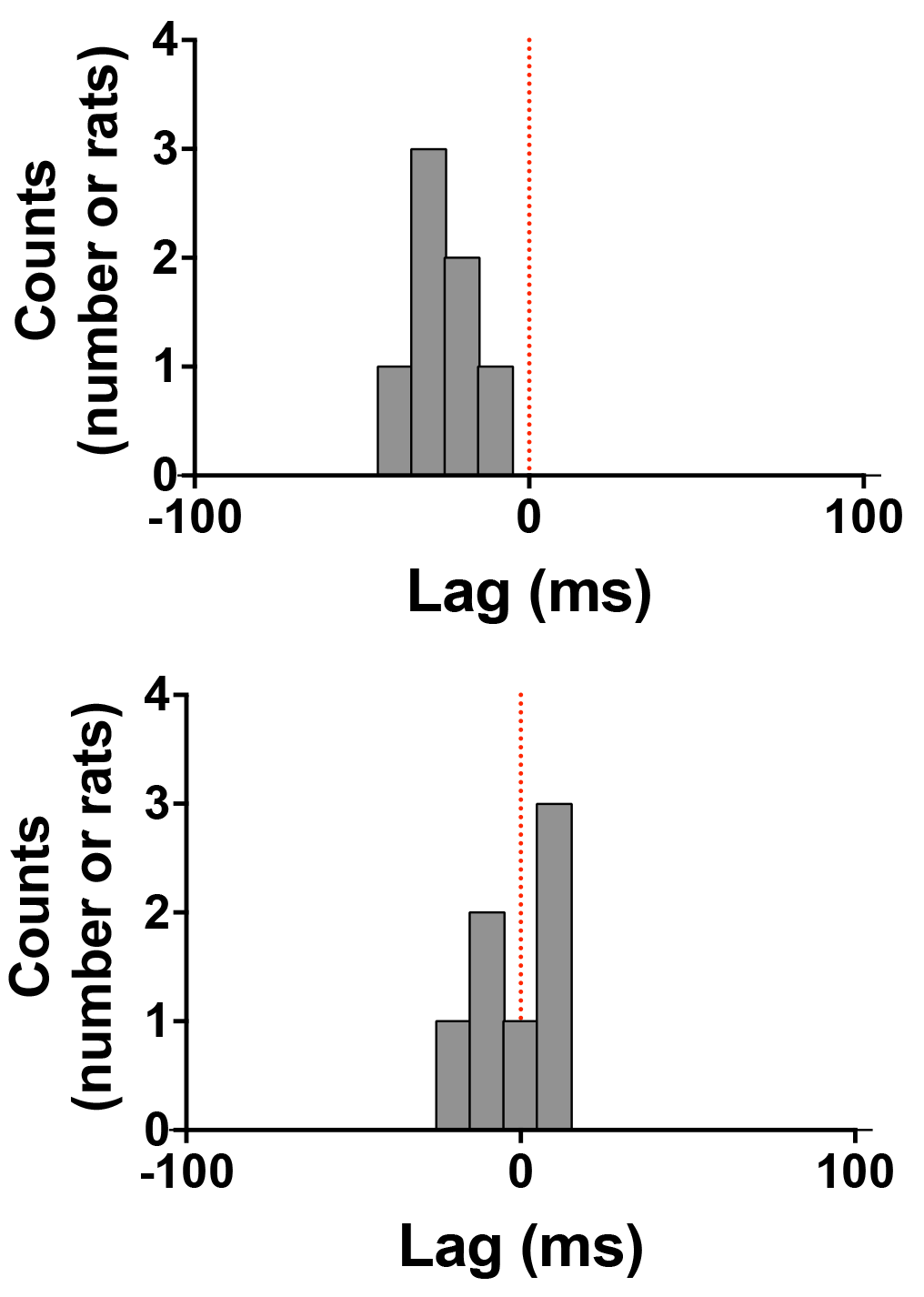Histograms of peak lag times for several rats (theta band top, gamma band bottom).

### Code

The Matlab code for the toolbox can be found here: LFP_Analysis
The phase locking and spike field coherence code is based on the methods described by Rutishauser et al..

1. Vagus Nerve Stimulation Alters Phase Synchrony of the Anterior Cingulate Cortex and Facilitates Decision Making in Rats
Scientific Reports, 2016, vol. 6, no. 35135.
2. Impairment of Cognitive Function by Chemotherapy: Association with the Disruption of Phase-Locking and Synchronization in Anterior Cingulate Cortex
Molecular Brain, 2015, vol. 8, no. 32.
3. Impairment of Decision Making and Disruption of Synchrony Between Basolateral Amygdala and Anterior Cingulate Cortex in the Maternally Separated Rat
Neurobiology of Learning and Memory, 2016, vol. 136, no. 32. pp. 74–85.